Next: 10.6.0.4 Forward Differencing Up: 10.6 Bump Mapping with Previous: 10.6.0.2 Approximating Bump Mapping   Contents

#### 10.6.0.3 Tangent Space

Recall that the bump map normalis formed by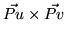. Assume that the surface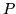is coincident with the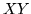plane and that changes in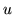and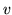correspond to changes in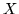and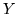, respectively. Then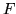can be substituted for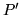, resulting in the following expression for the vector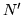: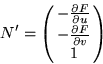In order to evaluate the lighting equation,must be normalized. If the displacements in the bump map are restricted to small values, however, the length ofwill be so close to one as to be approximated by one. Thenitself can be substituted for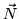without normalization. If the diffuse intensity component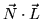of the lighting equation is evaluated with the value presented above for, the result is the following: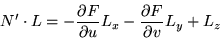(1)

This expression requires the surface to lie in theplane and that theandparameters change inand, respectively. Most surfaces, however, will have arbitrary locations and orientations in space. In order to use this simplification to perform bump mapping, the view direction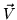, and light source direction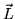are transformed into tangent space.

Tangent space has 3 axes,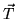,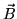and. The tangent vector,, is parallel to the direction of increasing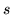on the surface. The normal vector,, is perpendicular to the surface. The binormal,, is perpendicular to bothand, and like, lies in the plane tangent to the surface. These vectors form a coordinate system that is attached to and varies over the surface.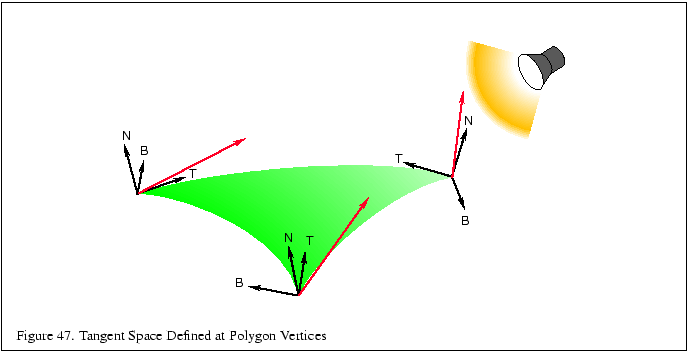The light source is transformed into tangent space at each vertex of the polygon. To find the tangent space vectors at a vertex, use the vertex normal forand find the tangent axisby finding the vector direction of increasingin the object's coordinate system. The direction of increasing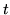may also be used. Findby computing the cross product ofand. These unit vectors form the transformation shown below: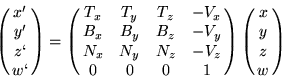(2)

This transformation brings coordinates into tangent space, where the plane tangent to the surface lies in the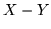plane, and the normal to the surface coincides with the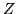axis. Note that the tangent space transformation varies for vertices representing a curved surface, and so this technique makes the approximation that curved surfaces are flat and the tangent space transformation is interpolated from vertex to vertex.Next: 10.6.0.4 Forward Differencing Up: 10.6 Bump Mapping with Previous: 10.6.0.2 Approximating Bump Mapping   Contents
2001-01-10